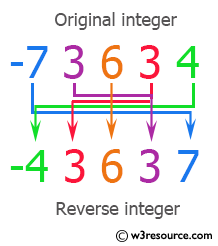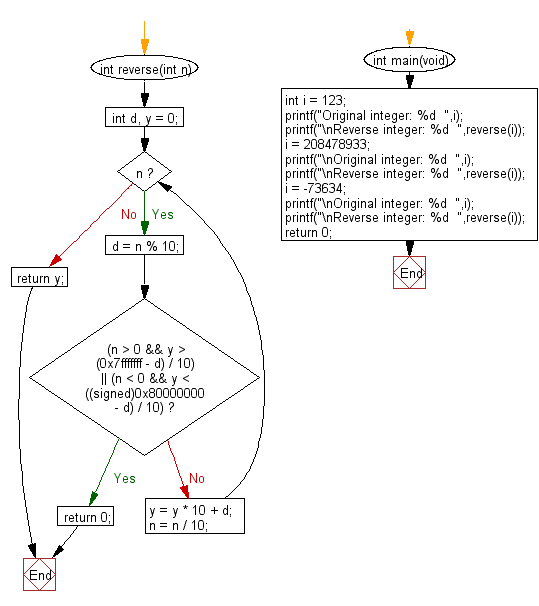﻿ C Program: Reverse the digits of a given integer - w3resource

# C Exercises: Reverse the digits of a given integer

## C Programming Mathematics: Exercise-1 with Solution

Write a C program to reverse the digits of a given integer.

Example:
Input:
i = 123
i = 208478933
i = -73634
Output:
Reverse integer: 321
Reverse integer: 339874802
Reverse integer: -43637

Pictorial Presentation:Sample Solution:

C Code:

``````#include <stdio.h>
int reverse(int n) {
int d, y = 0;
while (n) {
d = n % 10;
if ((n > 0 && y > (0x7fffffff - d) / 10) ||
(n < 0 && y < ((signed)0x80000000 - d) / 10)) {
return 0;
}
y = y * 10 + d;
n = n / 10;
}
return y;
}

int main(void)
{
int i = 123;
printf("Original integer: %d  ",i);
printf("\nReverse integer: %d  ",reverse(i));
i = 208478933;
printf("\nOriginal integer: %d  ",i);
printf("\nReverse integer: %d  ",reverse(i));
i = -73634;
printf("\nOriginal integer: %d  ",i);
printf("\nReverse integer: %d  ",reverse(i));
return 0;
}
```
```

Sample Output:

```Original integer: 123
Reverse integer: 321
Original integer: 208478933
Reverse integer: 339874802
Original integer: -73634
Reverse integer: -43637
```

Flowchart:C Programming Code Editor:

Improve this sample solution and post your code through Disqus.

What is the difficulty level of this exercise?

Test your Programming skills with w3resource's quiz.

﻿